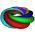## Thursday, October 18, 2012

### Standard Form of a Line

From my favorite mathwords.com

What is it that makes this one so tough?  Maybe the last sentence above?  Maybe the A>0 part?

-Mr. Morrissey

#### 1 comment:

1.I think the A > 0 part is difficult to remember because the choice between writing 2x - 7y = 5 or 7y - 2x = -5, or even -2x + 7y = -5 is arbitrary and not so important when you really think about it.

In my multivariable calculus class, we get credit for writing equations of planes either as 2x - 7y + 3z = 0 or -2x + 7y - 3z = 0. The distinction between these two forms (which are negatives of each other) is ultimately a trivial point compared to the bigger concept of lines and planes.

However, I know that one particular application of standard forms is so that computer algebra systems can easily check if two equations of a line are equivalent by reducing both equations to standard form and comparing coefficients.